Next: One-Dimensional Potentials Up: Stationary States Previous: Stationary States

Exercises

1. Monochromatic light with a wavelength of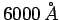passes through a fast shutter that opens for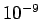sec. What is the subsequent spread in wavelengths of the no longer monochromatic light?

2. Calculate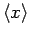,, and, as well as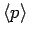,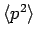, and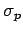, for the normalized wavefunction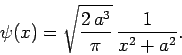Use these to find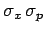. Note that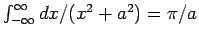.

3. Classically, if a particle is not observed then the probability of finding it in a one-dimensional box of length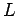, which extends fromto, is a constant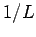per unit length. Show that the classical expectation value ofis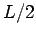, the expectation value of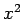is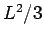, and the standard deviation ofis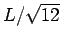.

4. Demonstrate that if a particle in a one-dimensional stationary state is bound then the expectation value of its momentum must be zero.

5. Suppose thatis complex. Obtain an expression forandfrom Schrödinger's equation. What does this tell us about a complex?

6.and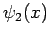are normalized eigenfunctions corresponding to the same eigenvalue. If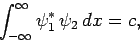where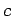is real, find normalized linear combinations of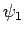and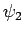which are orthogonal to (a), (b).

7. Demonstrate thatis an Hermitian operator. Find the Hermitian conjugate of.

8. An operator, corresponding to a physical quantity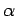, has two normalized eigenfunctionsand, with eigenvalues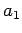and. An operator, corresponding to another physical quantity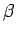, has normalized eigenfunctions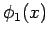and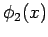, with eigenvalues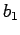and. The eigenfunctions are related via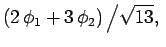is measured and the valueis obtained. Ifis then measured and thenagain, show that the probability of obtaininga second time is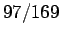.

9. Demonstrate that an operator which commutes with the Hamiltonian, and contains no explicit time dependence, has an expectation value which is constant in time.

10. For a certain system, the operator corresponding to the physical quantitydoes not commute with the Hamiltonian. It has eigenvaluesand, corresponding to properly normalized eigenfunctions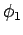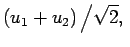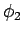where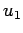andare properly normalized eigenfunctions of the Hamiltonian with eigenvaluesand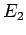. If the system is in the state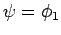at time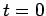, show that the expectation value ofat timeisNext: One-Dimensional Potentials Up: Stationary States Previous: Stationary States
Richard Fitzpatrick 2010-07-20